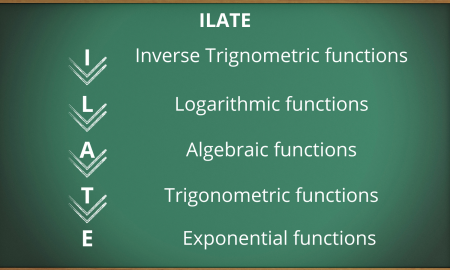GeeksforGeeks App
Open AppBrowser
Continue

## Related Articles

In Calculus, for every kind of function, there exists a formula to directly evaluate their integral. But what if we are required to figure out the integral of a product of two functions? Integration by parts is the answer to this. The formula for Integration by parts is given as:

∫uv dx = u∫v dx − ∫((du/dx)∫v dx) dx

Where:

• u is the first function of x: u(x)
• v is the second function of x: v(x)

As the name suggests, in Integration by parts, we first check which kind of two functions compose the given expression to be integrated. Then we check their precedence according to a simple rule called the ILATE rule. This rule helps us determine which function should be treated as the first function of x that is u(x) and which function should be referred to as the second function that is v(x).

### ILATE Rule

ILATE is an acronym for the type of functions listed below. Out of the given two functions, the function which is upper in the hierarchy comparatively is treated as the first function and the other one as the second function.### Steps after finding u(x) and v(x)

1. Differentiate u(x) with respect to x that is, evaluate du/dx.
2. Integrate v(x) with respect to x that is, evaluate ∫v dx.
3. Use the results obtained in step 1 & 2 in the formula: ∫uv dx = u∫v dx − ∫((du/dx)∫v dx) dx

### Examples

Find the integration of the following expression by using the by Parts rule:

Question 1. ∫ ex x dx

Solution:

Choosing u and v,

u = x and v = ex

Differentiating u:

u'(x) = d(u)/dx

= d(x)/dx

= 1

Using the formula,

∫ ex x dx = x ∫ex dx − ∫1 (∫ ex dx) dx

= xex − ex + C

= ex(x − 1) + C

Question 2. ∫ x sin x dx

Solution:

Choosing u and v,

u = x and v = sin x

Differentiating u:

u'(x) = d(u)/dx

= d(x)/dx

= 1

Using the formula,

∫ x sin x dx = x ∫sin x dx − ∫1 ∫(sin x dx) dx

= − x cos x − ∫−cos x dx

= − x cos x + sin x + C

Question 3. ∫ ln x dx

Solution:

Since here is only one function, thus we need to assume the other function so that the integration by parts’ formula can be applied to it. Also, the questions having the logarithmic functions can be solved only by parts.

Choosing u and v,

u = ln x and v = 1

Differentiating u:

u'(x) = d(u)/dx

= d(ln x)/dx

= 1/x

Using the formula,

∫ ln x dx = ln x∫ 1 dx − ∫ 1/x ∫(1 dx) dx

= x ln x −  ∫(1/x) x  dx       [since, ∫ 1 dx = x ]

= x ln x − ∫ 1 dx

= x ln x − x + C

= x (ln x − 1) + C

Question 4. ∫ sin−1 x  dx

Solution:

Since here is only one function, thus we need to assume the other function so that the integration by parts’ formula can be applied to it.

Choosing u and v,

u = sin−1 x  and  v = 1

Differentiating u:

u'(x) = d(u)/dx

= d(sin−1 x )/dx

= 1/√(1 − x 2

Using the formula,

∫ sin−1 x dx = sin−1 x ∫ 1 dx  − ∫ 1/√(1 − x 2)  ∫(1 dx)  dx

= x sin−1 x  − ∫( x/√(1 − x 2 ) )dx

Here, assuming t = 1 − x 2 and on differentiating both sides,

dt = −2x dx

−dt/2 = x dx

∫ sin−1 x dx =  x sin−1 x  − ∫−(1/2√t ) dt

= x sin−1 x  + 1/2∫t−1/2 dt

= x sin−1 x + t1/2 + C

= x sin−1 x + √(1 − x2 ) + C

My Personal Notes arrow_drop_up
Related Tutorials